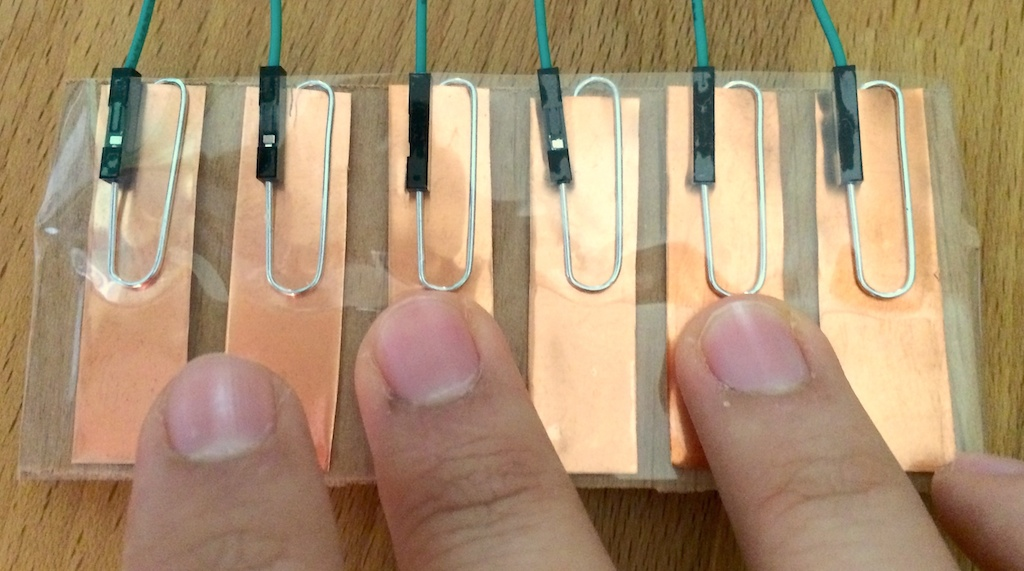Build this project at our next weekend-long Arduino workshop!# Capacitive Keyboard

The capacitive keyboard shows how to use capacitive sensing to handle six values at a time. Tapping the keys sends data to a Processing sketch that shows how much you’re pressing on the kays and plays “notes” based on your taps.

Requires minimal wiring skill; moderately complex programming.

## Hardware

You’ll need the following components:

• 6× 1 MΩ resistors.
• 6 paperclips and optionally copper foil to make a more solid terminal.
• 20 or so wires.
• 1 computer running Processing.

## Wiring

1. Build this circuit:2. Optional: Plug jumper wires into the ribbon cable and your Arduino as shown in this Adafruit tutorial. Try to match colors if you can; it will make for less (and eaiser) debugging.

## Software

2. Copy this code into a new Arduino sketch:

``````// Capacitive Keyboard + LED Matrix bubbles
// by J.D. Zamfirescu
// Last update: June 2014
// capacitive_keyboard.ino

#include <CapacitiveSensor.h>

const int numSensors = 6;
const int inputPins[] = {6, 7, 8, 9, 10, 11};

HT1632LEDMatrix matrix = HT1632LEDMatrix(DATA, WR, CS);
CapacitiveSensor *sensors;

void setup() {
for (int i = 0; i < numSensors; ++i) {
sensors[i] = new CapacitiveSensor(12, inputPins[i]);
}
Serial.begin(9600);
}

void loop() {
printTimings();

delay(20);
}

long timings[] = {0, 0, 0, 0, 0, 0};

for (int i = 0; i < numSensors; ++i) {
timings[i] = sensors[i]->capacitiveSensor(10);
}
}

void printTimings() {
for (int i = 0; i < numSensors; ++i) {
if (i != 0) {
Serial.print(",");
}
Serial.print(timings[i]);
}
Serial.println();
}
``````

5. Make a new Processing sketch with the following code:

``````// Circular Grapher
// by J.D. Zamfirescu
// Last update: June 2014
// circular_piano.pde

import processing.serial.*;

Serial port;
String serialInterface = "<your interface here>";

// for the graph
final int numSamples = 60;
final int numSources = 6;
int[][] values;
int[] hues = {0, 40, 60, 120, 240, 300};

// for the sound
import ddf.minim.*;
import ddf.minim.ugens.*;

Minim minim;
AudioOutput out;

// to make an Instrument we must define a class
// that implements the Instrument interface.
class SineInstrument implements Instrument
{
Oscil wave;
Line  ampEnv;

SineInstrument( float frequency )
{
// make a sine wave oscillator
// the amplitude is zero because
// we are going to patch a Line to it anyway
wave   = new Oscil( frequency, 0, Waves.SINE );
ampEnv = new Line();
ampEnv.patch( wave.amplitude );
}

// this is called by the sequencer when this instrument
// should start making sound. the duration is expressed in seconds.
void noteOn( float duration )
{
// start the amplitude envelope
ampEnv.activate( duration, 0.5f, 0 );
// attach the oscil to the output so it makes sound
wave.patch( out );
}

// this is called by the sequencer when the instrument should
// stop making sound
void noteOff()
{
wave.unpatch( out );
}
}

void setup() {
// for the graph
size(512, 512);
background(255);
port = new Serial(this, serialInterface, 9600);
values = new int[numSources][numSamples];
colorMode(HSB, 360, 100, 100);

// for the sound
minim = new Minim(this);

// use the getLineOut method of the Minim object to get an AudioOutput object
out = minim.getLineOut();
}

int ptr = 0;

// for the graph
void drawValues() {
background(0);
strokeWeight(4);
strokeCap(SQUARE);
for (int i = 0; i < numSamples; ++i) {
float startx = width/2 + width/8*cos(TWO_PI/numSamples * i);
float starty = height/2 + height/8*sin(TWO_PI/numSamples * i);
for (int source = 0; source < numSources; ++source) {
stroke(hues[source], 100, 100);
float x = startx + max(values[source][i] * width/120/numSources, 3) * cos(TWO_PI/numSamples * i);
float y = starty + max(values[source][i] * height/120/numSources, 3) * sin(TWO_PI/numSamples * i);
line(startx, starty, x, y);
// add some buffer between segments
startx = x + width/100 * cos(TWO_PI/numSamples * i);
starty = y + height/100 * sin(TWO_PI/numSamples * i);
}
}
}
// for the sound
int threshold = 30;
float[] notes = {
Frequency.ofPitch("C3").asHz(),
Frequency.ofPitch("D3").asHz(),
Frequency.ofPitch("E3").asHz(),
Frequency.ofPitch("F3").asHz(),
Frequency.ofPitch("G3").asHz(),
Frequency.ofPitch("A3").asHz(),
Frequency.ofPitch("B3").asHz()
};

void playNotes() {
int[] oldValues = new int[numSources];
int[] newValues = new int[numSources];
int oldPtr = (ptr + numSamples-2) % numSamples;
int newPtr = (oldPtr + 1) % numSamples;
for (int source = 0; source < numSources; ++source) {
oldValues[source] = values[source][oldPtr];
newValues[source] = values[source][newPtr];
if (newValues[source] > threshold && oldValues[source] < threshold) {
out.playNote(0.0, 1.0, new SineInstrument(notes[source]));
}
}
}

void handleData(int[] samples) {
for (int source = 0; source < samples.length; ++source) {
values[source][ptr] = samples[source];
}
ptr = (ptr + 1) % numSamples;
playNotes();
drawValues();
}

void draw() {
}

String s = null;
while ((s = port.readStringUntil('\n')) != null) {
String[] parts = s.substring(0, s.length()-2).split(",");

if (parts.length == numSources) {
int[] samples = new int[numSources];
for (int i = 0; i < numSources; ++i) {
samples[i] = int(parts[i]);
}
handleData(samples);
}
}
}
``````
6. Modify the `serialInterface` variable to point to the serial port your Arduino is connected on; you can find the port name in the lower right-hand corner of your Arduino IDE window.

7. Run the Processing sketch!

## Variations

1. Change the threshold value to make the keyboard more or less sensitive.

2. Add extra keys to the keyboard, and extra notes to the code.

3. Challenge: Modify the code so that the notes are louder or last longer the harder you press. (This challenge requires some advanced programming skill!)